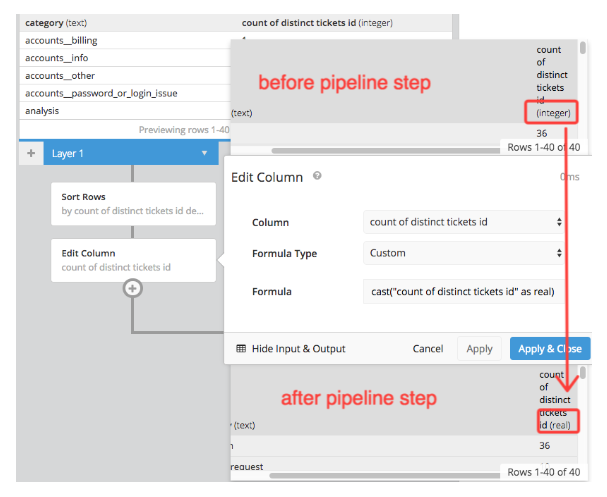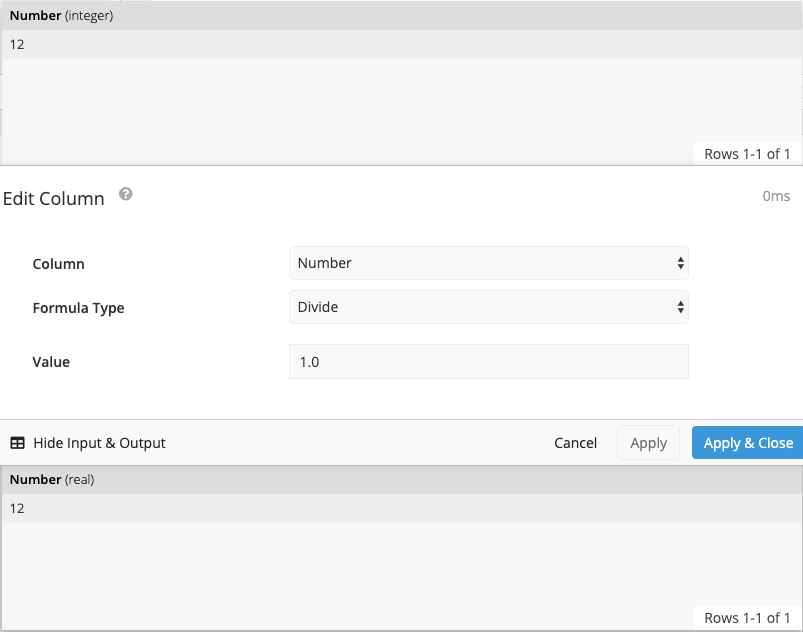# Change a data type in Data Explorer's Pipeline

The Chartio Data Pipeline allows you to utilize SQLite functions and syntax to manipulate your data. Please refer to the list of core functions that can be used in the Pipeline to learn more about the different options available and how they can be utilized to transform your data in Chartio.

One of these functions can be used to change a column’s data type. To do this, we can use either an Add Column or Edit Column step in the Pipeline and use a Custom formula. In this custom formula we will use the CAST expression so we can change the data type of a column.A CAST statement will look like the below example. It uses the form of specifying your column name and the use the word AS followed by a data type to specify the new data type the column should be converted over to.

``````cast("your_column_name" AS TYPE)
``````

In the example above, we changed the column’s data type from an integer to real. Changing our numeric column to real will enable us to use the column as part of calculations and get decimal precision in our results. Therefore, we used the following formula in our Edit Column step to change our column to real:

``````cast("count of distinct tickets id" as real)
``````

Another method that can be used in the data pipeline to convert an integer to a float is to divide the column by 1.0. Since we are dividing an integer column by a float the new values will be of float data type. Using a value of 1.0 will also preserve the original values of the dataset. In the below example we use an edit column pipeline step with the divide formula type.You can read more about the different data types in SQLite’s documentation.